Win up to 100% scholarship on Aakash BYJU'S JEE/NEET courses with ABNAT Win up to 100% scholarship on Aakash BYJU'S JEE/NEET courses with ABNAT

# JEE Main 2017 Chemistry Paper With Solutions (April 2)

JEE Main 2017 Paper with Solutions chemistry – Offline Exam is a perfect resource to crack the exams, which provide the gateway to some of the renowned institutions of the country. Due to excessive competition, students need to prepare well in advance. The solutions uploaded at BYJU’S will enable the students to divide and plan their resources in an optimal way. Practising these questions will make them understand the amount of preparation required to face the examination.
April 2nd – Chemistry

Question 1: Given C(graphite) + O2 (g) → CO2 (g) ; ∆r Ho = −393.5 kJ mol−1

H2 (g) + [1 / 2] O2 (g) → H2O (l) ;

r Ho= −285.8 kJ mol−1

CO2 (g) + 2H2O(l) → CH4 (g) + 2O2 (g) ;

r Ho = +890.3 kJ mol−1

Based on the above thermochemical equations, the value of ∆rHo at 298 K for the reaction C(graphite) + 2H2 (g) → CH4 (g) will be :

1) −74.8 kJ mol−1
2) −144.0 kJ mol−1
3) +74.8 kJ mol−1
4) +144.0 kJ mol−1

C(graphite) + O2 (g) → CO2 (g);

r Ho = –393.5 kJ mol–1 …(i)

H2 (g) + [1 / 2] O2 (g) → H2O (l) ;

r Ho = −285.8 kJ mol−1 …. (ii)

CO2 (g) + 2H2O(l) → CH4 (g) + 2O2 (g) ;

r Ho = +890.3 kJ mol−1 … (iii)

By applying the operation (i) + 2 × (ii) + (iii), we get

C(graphite) + 2H2(g) → CH4(g);

r Ho = –393.5 –285.8 × 2 + 890.3

= –74.8 kJ mol–1

Question 2: 1 gram of a carbonate (M2CO3) on treatment with excess HCl produces 0.01186 moles of CO2. The molar mass of M2CO3 in g mol−1 is :

1) 118.6
2) 11.86
3) 1186
4) 84.3

M2CO3 + 2HCl → 2MCl + H2O + CO2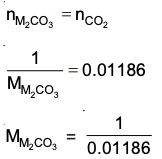= 84.3 g/mol

Question 3: ∆U is equal to :

2) Isothermal work
3) Isochoric work
4) Isobaric work

For adiabatic process, q = 0

As per 1st law of thermodynamics, ΔU = W.

Question 4: The Tyndall effect is observed only when the following conditions are satisfied :

(a) The diameter of the dispersed particles is much smaller than the wavelength of the light used.

(b) The diameter of the dispersed particle is not much smaller than the wavelength of the light used.

(c) The refractive indices of the dispersed phase and dispersion medium are almost similar in magnitude.

(d) The refractive indices of the dispersed phase and dispersion medium differ greatly in magnitude.

1) (a) and (c)
2) (b) and (c)
3) (a) and (d)
4) (b) and (d)

Answer: For Tyndall effect refractive index of dispersion phase and dispersion medium must differ significantly. Secondly, the size of the dispersed phase should not differ much from the wavelength used.

Question 5: A metal crystallises in a face centred cubic structure. If the edge length of its unit cell is ‘a’, the closest approach between two atoms in metallic crystal will be :

1) √2 a
2) a/√2
3) 2a
4) 2√2 a

In FCC, one of the faces is like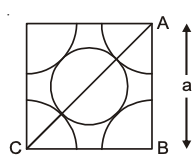By △ABC,

2a2 = 16r2

r2 = (1 / 8) a2

r = 1 / 2√2 a

Distance of closest approach = 2r = a / √2

Question 6: Given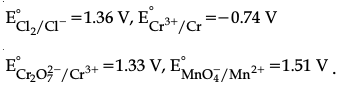Among the following, the strongest reducing agent is:

1) Cr3+
2) Cl
3) Cr
4) Mn2+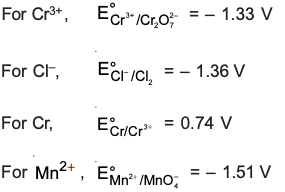Positive E° is for Cr, hence it is the strongest reducing agent.

Question 7: The freezing point of benzene decreases by 0.45oC when 0.2 g of acetic acid is added to 20 g of benzene. If acetic acid associates to form a dimer in benzene, percentage association of acetic acid in benzene will be : (Kf for benzene = 5.12 K kg mol−1)

1) 74.6%
2) 94.6%
3) 64.6%
4) 80.4%

0.45 = i (5.12) [(0.2 / 60) / 20] * 1000

i = 0.527

2CH3 COOH [CH3 COOH]2

(1 – α) α / 2

i = (1 – α) / 2

0.527 = 1 – (α / 2)

α / 2 = 0.473

α = 0.946

% association = 94.6%

Question 8: The radius of the second Bohr orbit for the hydrogen atom is : (Planck’s Constant. h = 6.6262 × 10−34 Js; the mass of electron = 9.1091 × 10−31 kg; charge of electron e = 1.60210 × 10−19 C; permittivity of vacuum ∈0 = 8.854185 × 10−12 kg−1m−3A2)

1) 0.529 Å
2) 2.12 Å
3) 1.65 Å
4) 4.76 Å

r = a0 (n2 / Z)

= 0.529 × 4

= 2.12 Å

Question 9: Two reactions R1 and R2 have identical pre-exponential factors. The activation energy of R1 exceeds that of R2 by 10 kJ mol−1. If k1 and k2 are rate constants for reactions R1 and R2 respectively at 300 K, then ln (k2/k1) is equal to (R = 8.314 J mol−1K−1)

1) 6
2) 4
3) 8
4) 12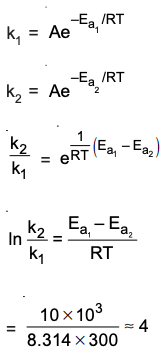Question 10: pKa of a weak acid (HA) and pKb of a weak base (BOH) are 3.2 and 3.4, respectively. The pH of their salt (AB) solution is :

1) 7.0
2) 1.0
3) 7.2
4) 6.9

pH = 7 + [(1 / 2) (pKa – pKb)]

= 7 + (1 / 2) [3.2 – 3.4]

= 6.9

Question 11: Both lithium and magnesium display several similar properties due to the diagonal relationship; however, the one which is incorrect, is :

1) both form nitrides
2) nitrates of both Li and Mg yield NO2 and O2 on heating
3) both form basic carbonates
4) both form soluble bicarbonates

Mg forms basic carbonate 3MgCO3 · Mg (OH)2 · 3H2O but no such basic carbonate is formed by Li.

Question 12: Which of the following species is not paramagnetic?

1) O2
2) B2
3) NO
4) CO

CO has 14 electrons (even) ∴ it is diamagnetic.

NO has 15e(odd) ∴ it is paramagnetic and has 1 unpaired electron in π * 2p molecular orbital.

B2 has 10e (even) but still paramagnetic and has two unpaired electrons in π * 2px and π * 2py (s-p mixing).

O2 has 16 e (even) but still paramagnetic and has two unpaired electrons in π * 2px and π * 2py molecular orbitals.

Question 13: Which of the following reactions is an example of a redox reaction?

1) XeF6 + H2O → XeOF4 + 2HF
2) XeF6 + 2H2O → XeO2 F2 + 4HF
3) XeF4 + O2 F2 → XeF6 + O2
4) XeF2 + PF5 → [XeF]+ PF6

Xe is oxidised from +4 (in XeF4) to +6 (in XeF6)

Oxygen is reduced from +1 (in O2F2) to zero (in O2)

Question 14: A water sample has ppm level concentration of following anions

F = 10; SO2-4 = 100; NO3 = 50

The anion/anions that make/makes the water sample unsuitable for drinking is/ are :

1) only F
2) only SO2-4
3) only NO3
4) both SO2-4 and NO3

The permissible limit of F in drinking water is up to 1 ppm.

Excess concentration of F > 10 ppm causes decay of bones.

Question 15: The group having isoelectronic species is :

1) O2, F, Na, Mg2+
2) O, F, Na+, Mg2+
3) O2−, F, Na+, Mg2+
4) O, F, Na, Mg+

Mg2+, Na+, O2– and F all have 10 electrons each.

Question 16: The products obtained when chlorine gas reacts with cold and dilute aqueous NaOH are :

1) Cl and ClO
2) Cl and ClO2
3) ClOand ClO3
4) ClO2 and ClO3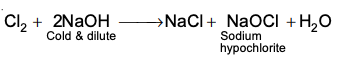Question 17: In the following reactions, ZnO is respectively acting as a/an :

(a) ZnO + Na2O → Na2ZnO2

(b) ZnO + CO2 → ZnCO3

1) acid and acid
2) acid and base
3) base and acid
4) base and base

In (a), ZnO acts as acidic oxide as Na2O is a basic oxide.

In (b), ZnO acts as basic oxide as CO2 is an acidic oxide.

Question 18: Sodium salt of an organic acid ‘X’ produces effervescence with conc. H2SO4. ‘X’ reacts with the acidified aqueous CaCl2 solution to give a white precipitate which decolourises the acidic solution of KMnO4. ‘X’ is :

1) CH3COONa
2) Na2C2O4
3) C6H5COONa
4) HCOONa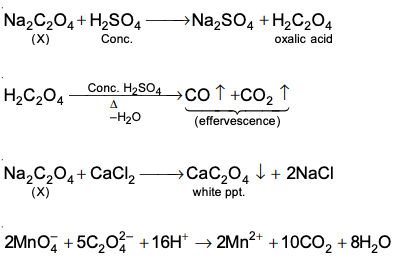Question 19: The most abundant elements by mass in the body of a healthy human adult are: Oxygen (61.4%); Carbon (22.9%), Hydrogen (10.0%); and Nitrogen (2.6%). The weight which a 75 kg person would gain if all 1H atoms are replaced by 2H atoms is :

1) 7.5 kg
2) 10 kg
3) 15 kg
4) 37.5 kg

Mass of hydrogen = (10/100 ) * 75 = 7.5 kg

Replacing 1H by 2H would replace 7.5 kg with 15 kg.

Net gain = 7.5 kg

Question 20: On treatment of 100 mL of 0.1 M solution of CoCl3.6H2O with excess AgNO3; 1.2 × 1022 ions are precipitated. The complex is :

1) [Co(H2O)6]Cl3
2) [Co(H2O)5Cl]Cl2.H2O
3) [Co(H2O)4Cl2]Cl.2H2O
4) [Co(H2O)3Cl3].3H2O

Millimoles of AgNO3 = [(1.2 x 1022) / (6 * 1023)] * 1000 = 20

Millimoles of CoCl3.6H2O = 0.1 × 100 = 10

Each mole of CoCl3.6H2O gives two chloride ions.

[Co(H2O)5Cl]Cl2.H2O

Question 21: Which of the following compounds will form a significant amount of meta product during the mono-nitration reaction?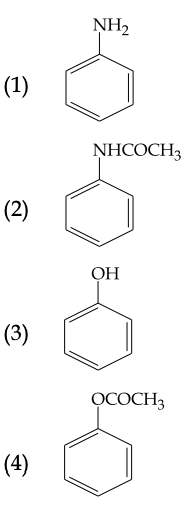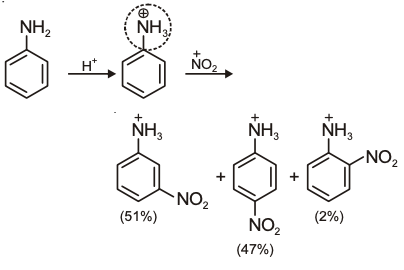Question 22: Which of the following, upon treatment with tert-BuONa followed by addition of bromine water, fails to decolourize the colour of bromine?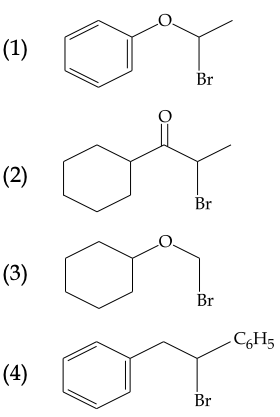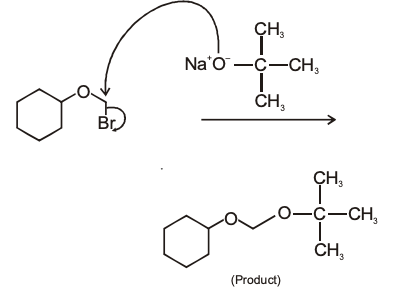Question 23: The formation of which of the following polymers involves hydrolysis reaction?

1) Nylon 6, 6
2) Terylene
3) Nylon 6
4) Bakelite

Caprolactam is hydrolysed to produce caproic acid which undergoes condensation to produce Nylon-6.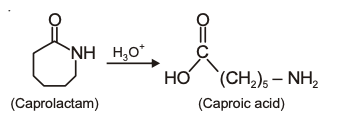Question 24: Which of the following molecules is the least resonance stabilized?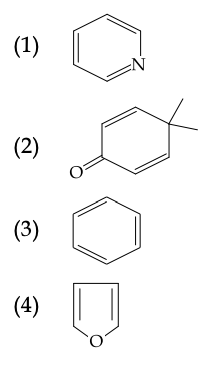However, all molecules given in options are stabilised by resonance but compound given in option (2) is least resonance stabilised (other three are aromatic)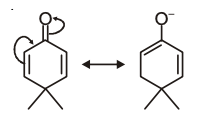Question 25: The increasing order of the reactivity of the following halides for the SN1 reaction is :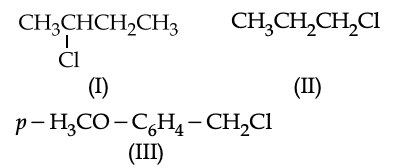1) (I) < (III) < (II)
2) (II) < (III) < (I)
3) (III) < (II) < (I)
4) (II) < (I) < (III)

Rate of SN1 reaction ∝ stability of carbocation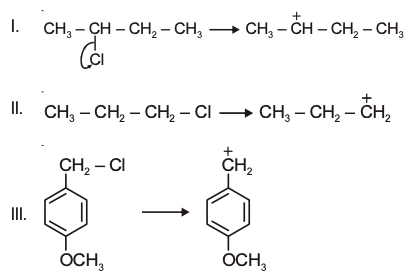So, II < I < III

Increase in the stability of carbocation and hence increase the reactivity of halides.

Question 26: The major product obtained in the following reaction is: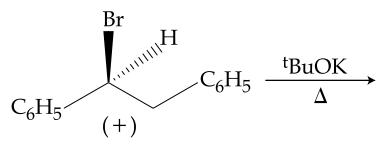1) (+) C6H5CH(OtBu)CH2C6H5
2) (−) C6H5CH(OtBu)CH2C6H5
3) (±) C6H5CH(OtBu)CH2C6H5
4) C6H5CH = CHC6H5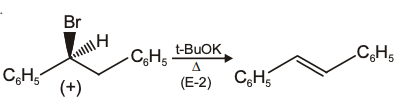Question 27: Which of the following compounds will behave as reducing sugar in an aqueous KOH solution?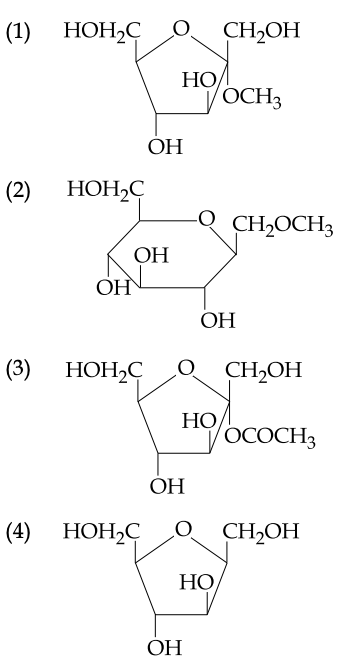Sugars in which there is free anomeric –OH group are reducing sugars.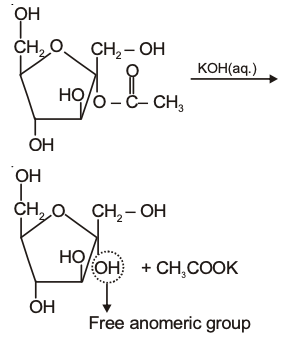Question 28: 3-Methyl-pent-2-ene on reaction with HBr in presence of peroxide forms an addition product. The number of possible stereoisomers for the product is :

1) Two
2) Four
3) Six
4) Zero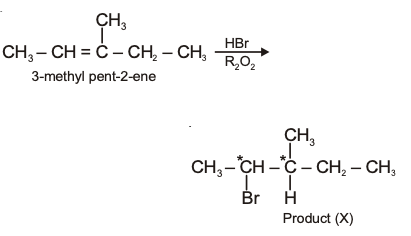Since the product (X) contains two chiral centres and it is unsymmetrical.

So, its total stereoisomers = 22 = 4.

Question 29: The correct sequence of reagents for the following conversion will be :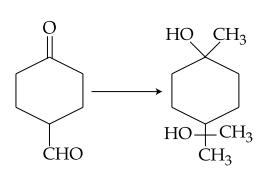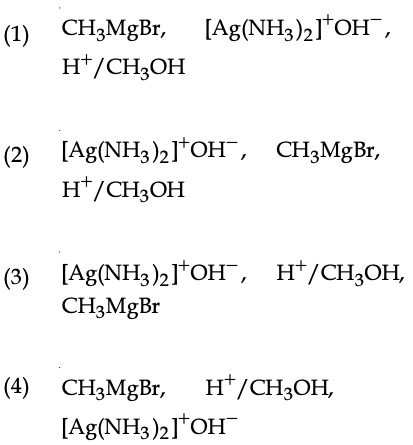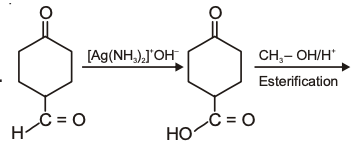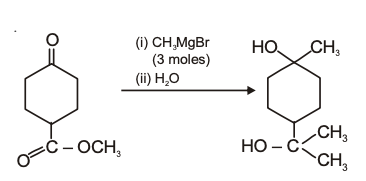Question 30: The major product obtained in the following reaction is :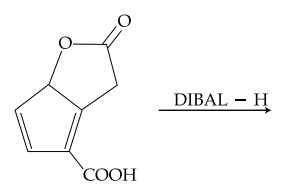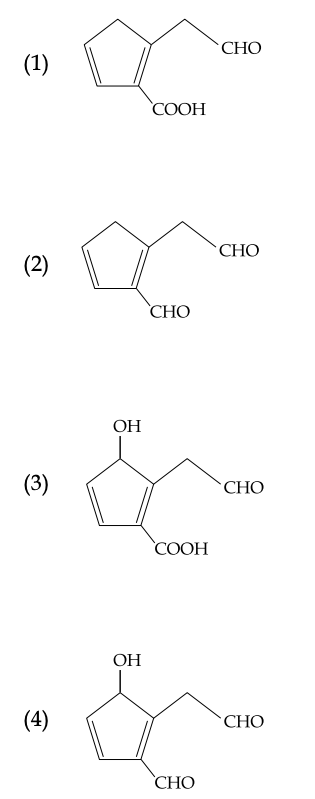DIBAL — H reduces esters and carboxylic acids into aldehydes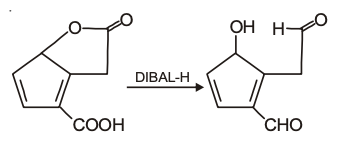## Video Lessons – April 2nd – Chemistry